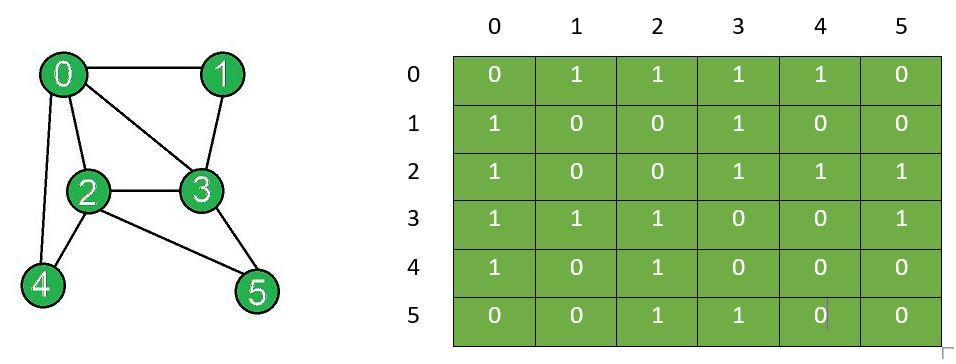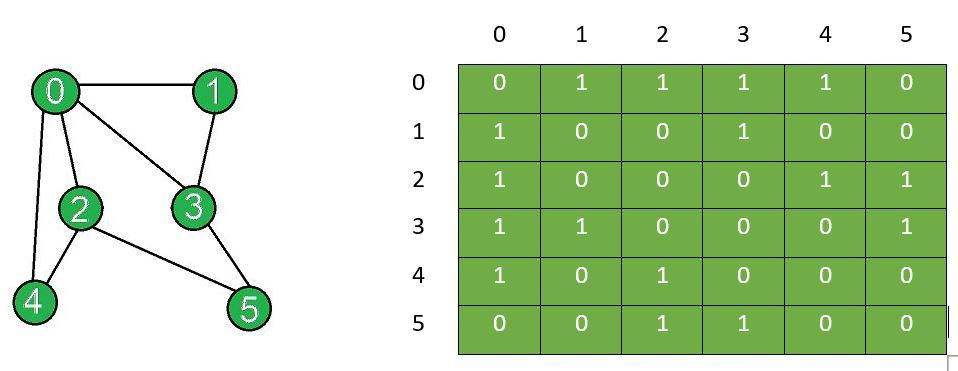Skip to content
Related Articles
Add and Remove Edge in Adjacency Matrix representation of a Graph
• Last Updated : 01 Jun, 2021

Prerequisites: Graph and its representations
Given an adjacency matrix g[][] of a graph consisting of N vertices, the task is to modify the matrix after insertion of all edges[] and removal of edge between vertices (X, Y). In an adjacency matrix, if an edge exists between vertices i and j of the graph, then g[i][j] = 1 and g[j][i] = 1. If no edge exists between these two vertices, then g[i][j] = 0 and g[j][i] = 0.
Examples:

Input: N = 6, Edges[] = {{0, 1}, {0, 2}, {0, 3}, {0, 4}, {1, 3}, {2, 3}, {2, 4}, {2, 5}, {3, 5}}, X = 2, Y = 3
Output:
Adjacency matrix after edge insertion:
0 1 1 1 1 0
1 0 0 1 0 0
1 0 0 1 1 1
1 1 1 0 0 1
1 0 1 0 0 0
0 0 1 1 0 0
Adjacency matrix after edge removal:
0 1 1 1 1 0
1 0 0 1 0 0
1 0 0 0 1 1
1 1 0 0 0 1
1 0 1 0 0 0
0 0 1 1 0 0
Explanation:
The graph and the corresponding adjacency matrix after insertion of edges:The graph after removal and adjacency matrix after removal of edge between vertex X and Y:Input: N = 6, Edges[] = {{0, 1}, {0, 2}, {0, 3}, {0, 4}, {1, 3}, {2, 3}, {2, 4}, {2, 5}, {3, 5}}, X = 3, Y = 5
Output:
Adjacency matrix after edge insertion:
0 1 1 1 1 0
1 0 0 1 0 0
1 0 0 1 1 1
1 1 1 0 0 1
1 0 1 0 0 0
0 0 1 1 0 0
Adjacency matrix after edge removal:
0 1 1 1 1 0
1 0 0 1 0 0
1 0 0 1 1 1
1 1 1 0 0 0
1 0 1 0 0 0
0 0 1 0 0 0

Approach:
Initialize a matrix of dimensions N x N and follow the steps below:

• Inserting an edge: To insert an edge between two vertices suppose i and j, set the corresponding values in the adjacency matrix equal to 1, i.e. g[i][j]=1 and g[j][i]=1 if both the vertices i and j exists.
• Removing an edge: To remove an edge between two vertices suppose i and j, set the corresponding values in the adjacency matrix equal to 0. That is, set g[i][j]=0 and g[j][i]=0 if both the vertices i and j exists.

Below is the implementation of the above approach:

## C++

 `// C++ program to add and remove edge``// in the adjacency matrix of a graph` `#include ``using` `namespace` `std;` `class` `Graph {``private``:``    ``// Number of vertices``    ``int` `n;` `    ``// Adjacency matrix``    ``int` `g;` `public``:``    ``// Constructor``    ``Graph(``int` `x)``    ``{``        ``n = x;` `        ``// Initializing each element of the``        ``// adjacency matrix to zero``        ``for` `(``int` `i = 0; i < n; i++) {``            ``for` `(``int` `j = 0; j < n; j++) {``                ``g[i][j] = 0;``            ``}``        ``}``    ``}` `    ``// Function to display adjacency matrix``    ``void` `displayAdjacencyMatrix()``    ``{``        ``// Displaying the 2D matrix``        ``for` `(``int` `i = 0; i < n; i++) {``            ``cout << ``"\n"``;``            ``for` `(``int` `j = 0; j < n; j++) {``                ``cout << ``" "` `<< g[i][j];``            ``}``        ``}``    ``}` `    ``// Function to update adjacency``    ``// matrix for edge insertion``    ``void` `addEdge(``int` `x, ``int` `y)``    ``{``        ``// Checks if the vertices``        ``// exist in the graph``        ``if` `((x < 0) || (x >= n)) {``            ``cout << ``"Vertex"` `<< x``                 ``<< ``" does not exist!"``;``        ``}``        ``if` `((y < 0) || (y >= n)) {``            ``cout << ``"Vertex"` `<< y``                 ``<< ``" does not exist!"``;``        ``}` `        ``// Checks if it is a self edge``        ``if` `(x == y) {``            ``cout << ``"Same Vertex!"``;``        ``}` `        ``else` `{``            ``// Insert edge``            ``g[y][x] = 1;``            ``g[x][y] = 1;``        ``}``    ``}` `    ``// Function to update adjacency``    ``// matrix for edge removal``    ``void` `removeEdge(``int` `x, ``int` `y)``    ``{``        ``// Checks if the vertices``        ``// exist in the graph``        ``if` `((x < 0) || (x >= n)) {``            ``cout << ``"Vertex"` `<< x``                 ``<< ``" does not exist!"``;``        ``}``        ``if` `((y < 0) || (y >= n)) {``            ``cout << ``"Vertex"` `<< y``                 ``<< ``" does not exist!"``;``        ``}` `        ``// Checks if it is a self edge``        ``if` `(x == y) {``            ``cout << ``"Same Vertex!"``;``        ``}` `        ``else` `{``            ``// Remove edge``            ``g[y][x] = 0;``            ``g[x][y] = 0;``        ``}``    ``}``};` `// Driver Code``int` `main()``{``    ``int` `N = 6, X = 2, Y = 3;` `    ``Graph obj(N);` `    ``// Adding edges to the graph``    ``obj.addEdge(0, 1);``    ``obj.addEdge(0, 2);``    ``obj.addEdge(0, 3);``    ``obj.addEdge(0, 4);``    ``obj.addEdge(1, 3);``    ``obj.addEdge(2, 3);``    ``obj.addEdge(2, 4);``    ``obj.addEdge(2, 5);``    ``obj.addEdge(3, 5);` `    ``cout << ``"Adjacency matrix after"``         ``<< ``" edge insertions:\n"``;``    ``obj.displayAdjacencyMatrix();` `    ``obj.removeEdge(X, Y);` `    ``cout << ``"\nAdjacency matrix after"``         ``<< ``" edge removal:\n"``;``    ``obj.displayAdjacencyMatrix();` `    ``return` `0;``}`

## Java

 `// Java program to add and remove edge``// in the adjacency matrix of a graph` `class` `Graph {` `    ``// Number of vertices``    ``private` `int` `n;` `    ``// Adjacency matrix``    ``private` `int``[][] g = ``new` `int``[``10``][``10``];` `    ``// Constructor``    ``Graph(``int` `x)``    ``{``        ``this``.n = x;` `        ``// Initializing each element of the``        ``// adjacency matrix to zero``        ``for` `(``int` `i = ``0``; i < n; ++i) {``            ``for` `(``int` `j = ``0``; j < n; ++j) {``                ``g[i][j] = ``0``;``            ``}``        ``}``    ``}` `    ``// Function to display adjacency matrix``    ``public` `void` `displayAdjacencyMatrix()``    ``{``        ``// Displaying the 2D matrix``        ``for` `(``int` `i = ``0``; i < n; ++i) {``            ``System.out.println();``            ``for` `(``int` `j = ``0``; j < n; ++j) {``                ``System.out.print(``" "` `+ g[i][j]);``            ``}``        ``}` `        ``System.out.println();``    ``}` `    ``// Function to update adjacency``    ``// matrix for edge insertion``    ``public` `void` `addEdge(``int` `x, ``int` `y)``    ``{``        ``// Checks if the vertices exists``        ``if` `((x < ``0``) || (x >= n)) {``            ``System.out.printf(``"Vertex "` `+ x``                              ``+ ``" does not exist!"``);``        ``}``        ``if` `((y < ``0``) || (y >= n)) {``            ``System.out.printf(``"Vertex "` `+ y``                              ``+ ``" does not exist!"``);``        ``}` `        ``// Checks if it is a self edge``        ``if` `(x == y) {``            ``System.out.println(``"Same Vertex!"``);``        ``}` `        ``else` `{``            ``// Insert edge``            ``g[y][x] = ``1``;``            ``g[x][y] = ``1``;``        ``}``    ``}` `    ``// Function to update adjacency``    ``// matrix for edge removal``    ``public` `void` `removeEdge(``int` `x, ``int` `y)``    ``{``        ``// Checks if the vertices exists``        ``if` `((x < ``0``) || (x >= n)) {``            ``System.out.printf(``"Vertex "` `+ x``                              ``+ ``" does not exist!"``);``        ``}``        ``if` `((y < ``0``) || (y >= n)) {``            ``System.out.printf(``"Vertex "` `+ y``                              ``+ ``" does not exist!"``);``        ``}` `        ``// Checks if it is a self edge``        ``if` `(x == y) {``            ``System.out.println(``"Same Vertex!"``);``        ``}` `        ``else` `{``            ``// Remove edge``            ``g[y][x] = ``0``;``            ``g[x][y] = ``0``;``        ``}``    ``}``}` `// Driver Code``class` `Main {``    ``public` `static` `void` `main(String[] args)``    ``{` `        ``int` `N = ``6``, X = ``2``, Y = ``3``;``        ``Graph obj = ``new` `Graph(N);` `        ``// Inserting edges``        ``obj.addEdge(``0``, ``1``);``        ``obj.addEdge(``0``, ``2``);``        ``obj.addEdge(``0``, ``3``);``        ``obj.addEdge(``0``, ``4``);``        ``obj.addEdge(``1``, ``3``);``        ``obj.addEdge(``2``, ``3``);``        ``obj.addEdge(``2``, ``4``);``        ``obj.addEdge(``2``, ``5``);``        ``obj.addEdge(``3``, ``5``);` `        ``System.out.println(``"Adjacency matrix after"``                           ``+ ``" edge insertions:"``);``        ``obj.displayAdjacencyMatrix();` `        ``obj.removeEdge(``2``, ``3``);` `        ``System.out.println(``"\nAdjacency matrix after"``                           ``+ ``" edge removal:"``);``        ``obj.displayAdjacencyMatrix();``    ``}``}`

## Python3

 `# Python3 program to add and remove edge``# in adjacency matrix representation of a graph``    ` `class` `Graph:``    ` `    ``# Number of vertices``    ``__n ``=` `0``    ` `    ``# Adjacency matrix``    ``__g ``=` `[[``0` `for` `x ``in` `range``(``10``)]``              ``for` `y ``in` `range``(``10``)]``        ` `    ``# Constructor``    ``def` `__init__(``self``, x):``        ``self``.__n ``=` `x``    ` `        ``# Initializing each element of``        ``# the adjacency matrix to zero``        ``for` `i ``in` `range``(``0``, ``self``.__n):``            ``for` `j ``in` `range``(``0``, ``self``.__n):``                ``self``.__g[i][j] ``=` `0``                ` `    ``# Function to display adjacency matrix            ``    ``def` `displayAdjacencyMatrix(``self``):``        ` `        ``# Displaying the 2D matrix``        ``for` `i ``in` `range``(``0``, ``self``.__n):``            ``print``()``            ``for` `j ``in` `range``(``0``, ``self``.__n):``                ``print``("``", self.__g[i][j], end = "``")``    ` `    ``# Function to update adjacency``    ``# matrix for edge insertion            ``    ``def` `addEdge(``self``, x, y):``        ` `        ``# Checks if the vertices``        ``# exist in the graph``        ``if` `(x < ``0``) ``or` `(x >``=` `self``.__n):``            ``print``(``"Vertex {} does not exist!"``.``format``(x))``        ``if` `(y < ``0``) ``or` `(y >``=` `self``.__n):``            ``print``(``"Vertex {} does not exist!"``.``format``(y))``            ` `        ``# Checks if it is a self edge``        ``if``(x ``=``=` `y):``            ``print``(``"Same Vertex!"``)` `        ``else``:``            ` `            ``# Adding edge between the vertices``            ``self``.__g[y][x] ``=` `1``            ``self``.__g[x][y] ``=` `1``    ` `    ``# Function to update adjacency``    ``# matrix for edge removal        ``    ``def` `removeEdge(``self``, x, y):``        ` `        ``# Checks if the vertices``        ``# exist in the graph``        ``if` `(x < ``0``) ``or` `(x >``=` `self``.__n):``            ``print``(``"Vertex {} does not exist!"``.``format``(x))``        ``if` `(y < ``0``) ``or` `(y >``=` `self``.__n):``            ``print``(``"Vertex {} does not exist!"``.``format``(y))``            ` `        ``# Checks if it is a self edge``        ``if``(x ``=``=` `y):``            ``print``(``"Same Vertex!"``)` `        ``else``:``            ` `            ``# Remove edge from between``            ``# the vertices``            ``self``.__g[y][x] ``=` `0``            ``self``.__g[x][y] ``=` `0` `# Driver code   ` `# Creating an object of class Graph``obj ``=` `Graph(``6``);``        ` `# Adding edges to the graph``obj.addEdge(``0``, ``1``)``obj.addEdge(``0``, ``2``)``obj.addEdge(``0``, ``3``)``obj.addEdge(``0``, ``4``)``obj.addEdge(``1``, ``3``)``obj.addEdge(``2``, ``3``)``obj.addEdge(``2``, ``4``)``obj.addEdge(``2``, ``5``)``obj.addEdge(``3``, ``5``)``    ` `# Edges added to the adjacency matrix``print``(``"Adjacency matrix after "``      ``"edge insertions:\n"``)``obj.displayAdjacencyMatrix();``        ` `# Removing the edge between vertices``# "2" and "3" from the graph``obj.removeEdge(``2``, ``3``);``    ` `# The adjacency matrix after``# removing the edge``print``(``"\nAdjacency matrix after "``      ``"edge removal:\n"``)``obj.displayAdjacencyMatrix();` `# This code is contributed by amarjeet_singh`

## C#

 `// C# program to add and remove edge``// in adjacency matrix representation``// of a graph``using` `System;``    ` `class` `Graph{``    ` `// Number of vertices``private` `int` `n;` `// Adjacency matrix``private` `int``[,] g = ``new` `int``[10, 10];` `// Constructor``public` `Graph(``int` `x)``{``    ``this``.n = x;` `    ``// Initializing each element of``    ``// the adjacency matrix to zero``    ``for``(``int` `i = 0; i < n; ++i)``    ``{``        ``for``(``int` `j = 0; j < n; ++j)``        ``{``            ``g[i, j] = 0;``        ``}``    ``}``}` `// Function to display adjacency matrix``public` `void` `displayAdjacencyMatrix()``{``    ` `    ``// Displaying the 2D matrix``    ``for``(``int` `i = 0; i < n; ++i)``    ``{``        ``Console.WriteLine();``        ``for``(``int` `j = 0; j < n; ++j)``        ``{``            ``Console.Write(``" "` `+ g[i, j]);``        ``}``    ``}``}` `// Function to update adjacency``// matrix for edge insertion``public` `void` `addEdge(``int` `x, ``int` `y)``{``    ` `    ``// Checks if the vertices exist``    ``// in the graph``    ``if` `((x < 0) || (x >= n))``    ``{``        ``Console.WriteLine(``"Vertex {0} does "` `+``                          ``"not exist!"``, x);``    ``}``    ``if` `((y < 0) || (y >= n))``    ``{``        ``Console.WriteLine(``"Vertex {0} does "` `+``                          ``"not exist!"``, y);``    ``}` `    ``// Checks if it is a self edge``    ``if` `(x == y)``    ``{``        ``Console.WriteLine(``"Same Vertex!"``);``    ``}``    ` `    ``else``    ``{``        ` `        ``// Adding edge between the vertices``        ``g[y, x] = 1;``        ``g[x, y] = 1;``    ``}``}` `// Function to update adjacency``// matrix for edge removal``public` `void` `removeEdge(``int` `x, ``int` `y)``{``    ` `    ``// Checks if the vertices exist``    ``// in the graph``    ``if` `((x < 0) || (x >= n))``    ``{``        ``Console.WriteLine(``"Vertex {0} does"` `+``                          ``"not exist!"``, x);``    ``}``    ``if` `((y < 0) || (y >= n))``    ``{``        ``Console.WriteLine(``"Vertex {0} does"` `+``                          ``"not exist!"``, y);``    ``}` `    ``// Checks if it is a self edge``    ``if` `(x == y)``    ``{``        ``Console.WriteLine(``"Same Vertex!"``);``    ``}``    ` `    ``else``    ``{``        ` `        ``// Remove edge from between``        ``// the vertices``        ``g[y, x] = 0;``        ``g[x, y] = 0;``    ``}``}``}``    ` `class` `GFG{``    ` `// Driver code``public` `static` `void` `Main(String[] args)``{``    ` `    ``// Creating an object of class Graph``    ``Graph obj = ``new` `Graph(6);` `    ``// Adding edges to the graph``    ``obj.addEdge(0, 1);``    ``obj.addEdge(0, 2);``    ``obj.addEdge(0, 3);``    ``obj.addEdge(0, 4);``    ``obj.addEdge(1, 3);``    ``obj.addEdge(2, 3);``    ``obj.addEdge(2, 4);``    ``obj.addEdge(2, 5);``    ``obj.addEdge(3, 5);` `    ``// Edges added to the adjacency matrix``    ``Console.WriteLine(``"Adjacency matrix after "` `+``                      ``"edge insertions:\n"``);``    ``obj.displayAdjacencyMatrix();` `    ``// Removing the edge between vertices``    ``// "2" and "3" from the graph``    ``obj.removeEdge(2, 3);``    ` `    ``// The adjacency matrix after``    ``// removing the edge``    ``Console.WriteLine(``"\nAdjacency matrix after "` `+``                      ``"edge removal:"``);``    ``obj.displayAdjacencyMatrix();``}``}` `// This code is contributed by amarjeet_singh`
Output:
```Adjacency matrix after edge insertions:

0 1 1 1 1 0
1 0 0 1 0 0
1 0 0 1 1 1
1 1 1 0 0 1
1 0 1 0 0 0
0 0 1 1 0 0
Adjacency matrix after edge removal:

0 1 1 1 1 0
1 0 0 1 0 0
1 0 0 0 1 1
1 1 0 0 0 1
1 0 1 0 0 0
0 0 1 1 0 0```

Time Complexity: Insertion and Deletion of an edge requires O(1) complexity while it takes O(N2) to display the adjacency matrix.
Auxiliary Space: O(N2)

Attention reader! Don’t stop learning now. Get hold of all the important DSA concepts with the DSA Self Paced Course at a student-friendly price and become industry ready.  To complete your preparation from learning a language to DS Algo and many more,  please refer Complete Interview Preparation Course.

In case you wish to attend live classes with industry experts, please refer DSA Live Classes

My Personal Notes arrow_drop_up